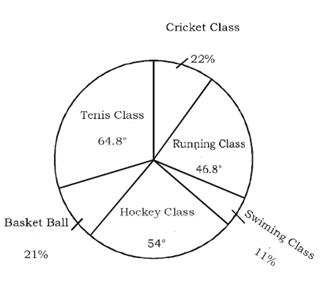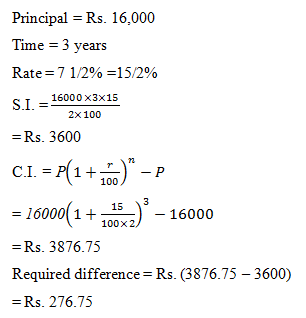# SSC CGL 2018 Practice Test Papers | Quantitative Aptitude (Day-38)

Dear Aspirants, Here we have given the Important SSC CGL Exam 2018 Practice Test Papers. Candidates those who are preparing for SSC CGL 2018 can practice these questions to get more confidence to Crack SSC CGL 2018 Examination.

[WpProQuiz 3304]

Click “Start Quiz” to attend these Questions and view Explanation

1) A man takes 3 hours 45 minutes to row a boat 15 km downstream of a river and 2 hours 30 minutes to cover a distance of 5 km upstream. Find the speed of the river current in km/hr.

a) 1 km/hr

b) 3km/hr

c) 5 km/hr

d) 9 km/hr

2) In a right angled isosceles triangle ABC, find the value of sinA + sinB + sinC.

a) 1-√2

b) 1 +√2

c) √2 -1

d) 1+√3

3) The average of 50 numbers is 42. If two numbers, 64 and 46 are discarded, the average of remaining number is

a) 43.5

b) 41.67

c) 45

d) 40

4) In the adjoining diagram, ABCD is a rectangle with AE = EF = FB. What is the ratio of the area of the triangle BCF and that of the rectangle?

a) 1 : 4

b) 1 : 6

c) 2 : 5

d) 2 : 3

5) A began a business with Rs. 1500 and B joined with Rs.2000 after few months. When did B Join, if the profit at the end of the year where divided in the ratio 3 : 2?

a) After 7 months

b) After 8 months

c) After 4 months

d) After 6 months

6) A lent Rs. 16000 on simple interest for 3 years at 7 ½ % p.a. How much more should have A gained, had A given it at compound interest at the same rate and for the same time?

a) Rs. 229.90

b) Rs. 299.20

c) Rs. 276.75

d) Rs. 292.20

(7-10)Study the pie–chart carefully and answer the questions based on it.Percentage of student enrolled in different game classes in a school

Total number of student = 3600

7) The number of student enrolled in cricket classes is what percent of that in Basket Ball classes?

a) 101.45%

b) 104.76%

c) 113.84%

d) 110.28%

8) What is the total number of student enrolled in swimming class and running class together?

a) 684

b) 846

c) 648

d) 864

9) How many students are enrolled in Hockey classes?

a) 540

b) 480

c) 450

d) 520

10) What is the ratio of the number of students enrolled in Tennis and Basket ball classes together to the number of students enrolled in running classes?

a) 3 : 1

b) 4 : 7

c) 7 : 5

d) 3 : 5

Downstream speed= 15/(3  ¾) = 4 km/hr

Upstream speed = 5/(2 ½) = 2 km/hr

Speed of River= ½(4-2) = 1 km/hr

In right angle isosceles triangle, angle will be 45º, 45º and 90º.

sinA + sinB + sinC = sin45º + sin45º + sin90º

= 1/√2 + 1/√2 + 1

= 1+√2

Average of 50 numbers = 42

Sum of 50 numbers = 50×42 = 2100

Sum of remaining numbers = 2100 – 64 – 46 = 2000

Average of remaining numbers = 2000/48 = 41.67

Area of triangle = ½ × Base × Height

Area of triangle CEF =1/2 × BC × EF

=1/2 ×BC× AB/3

= AB×BC/6

The area of ABCD = AB × BC

Required ratio =AB×BC/6: AB × BC=1:6

Ratio of profit = 3 : 2

So, ratio of investment = 3 :2

Total investment of A= 1500 × 12

So, total investment of B= 1500 × 12 ×2/3×1/ 2000 = 6

So, B joined after = 12 – 6 = 6 monthsRequired percentage = 22/21 × 100 = 104.76%

Student enrolled in Swimming class = 11/100 × 3600 = 396

Student enrolled in Running class = 46.8/360× 3600 = 468

Total number of students enrolled = 396 + 468 = 864